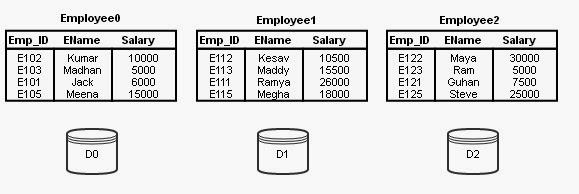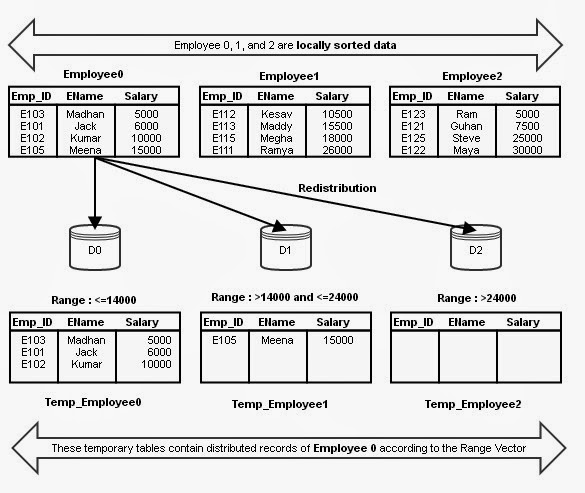Advanced Database Management System - Tutorials and Notes: Parallel Database - Parallel External Sort-Merge Technique

## Parallel External Sort-Merge

#### Assumptions:

Assume n processors, P0, P1, …, Pn-1 and n disks D0, D1, …, Dn-1.
Disk Di is associated with Processor Pi.
Relation R is partitioned into R0, R1, …, Rn-1 using Round-robin technique or Hash Partitioning technique or Range Partitioning technique (partitioned on any attribute)

#### Objective:

Our objective is to sort a relation (table) Ri on an attribute A in parallel where R resides on n disks.

#### Steps:

Step 1: Sort the relation partition Ri which is stored on disk Di on the sorting attribute of the query.
Step 2: Identify a range partition vector v and range partition every Ri into processors, P0, P1, …, Pn-1 using vector v.
Step 3: Each processor Pi performs a merge on the incoming range partitioned data from every other processors (The data are actually transferred in order. That is, all processors send first partition into P0, then all processors sends second partition into P1, and so on).
Step 4: Finally, concatenate all the sorted data from different processors to get the final result.

#### Point to note:

Range partition must be done using a good range-partitioning vector. Otherwise, skew might be the problem.

### Example:

Let us explain the above said process with simple example. Consider the following relation schema Employee;
Employee (Emp_ID, EName, Salary)
Assume that relation Employee is permanently partitioned using Round-robin technique into 3 disks D0, D1, and D2 which are associated with processors P0, P1, and P2. At processors P0, P1, and P2, the relations are named Employee0, Employee1 and Employee2 respectively. This initial state is given in Figure 1.Figure 1 - Partitioned Employee

Assume that the following sorting query is initiated.
SELECT * FROM Employee ORDER BY Salary;
As already said, the table Employee is not partitioned on the sorting attribute Salary. Then, the Parallel External Sort-Merge technique works as follows;

#### Step 1:

Sort the data stored in every partition (every disk) using the ordering attribute Salary. (Sorting of data in every partition is done temporarily). At this stage every Employeei contains salary values of range minimum to maximum. The partitions sorted in ascending order is shown below, in Figure 2.Figure 2 - Partitioned Employee in ascending order on Salary attribute

#### Step 2:

We have to identify a range vector v on the Salary attribute. The range vector is of the form v[v0, v1, …, vn-2]. For our example, let us assume the following range vector;
v[14000, 24000]
This range vector represents 3 ranges, range 0 (14000 and less), range 1 (14001 to 24000) and range 2 (24001 and more).
Redistribute every partition (Employee0, Employee1 and Employee2) using these range vectors into 3 disks temporarily. What would be the status of Temp_Employee 0, 1, and 2 after distributing Employee 0 is given in Figure 3.Figure 3 - Distribution of Employee 0 according to the range vector

#### Step 3:

Actually, the above said distribution is executed at all processors in parallel such that processors P0, P1, and P2 are sending the first partition of Employee 0, 1, and 2 to disk 0. Upon receiving the records from various partitions, the receiving processor P0 merges the sorted data. This is shown in Figure 4.Figure 4 - Merging data of range <=14000 at D0 from all the disks

The above said process is done at all processors for different partitions. The final version of Temp_Employee 0, 1, and 2 are shown in Figure 5.Figure 5 - Status of Temp_Employee 0, 1, and 2 after distributing all ranges from all disks

#### Step 4:

The final concatenation of sorted data from all the disks is trivial.

## Featured Content

### Multiple choice questions in Natural Language Processing Home

MCQ in Natural Language Processing, Quiz questions with answers in NLP, Top interview questions in NLP with answers Multiple Choice Que...